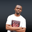Related Tags

php
operators
webdevelopment
communitycreator

# Difference between the (=), (==), and (===) operators in PHPNDUKWE CHIDERA K.

Operators in PHP are of different types, such as:

• Arithmetic
• Assignment
• Comparison
• Increment/Decrement
• Logical
• String
• Array
• Conditional assignment operators

These operator types have a list of different operators which have different functions. In this shot, we will look at some operators in the assignment and comparison types.

### The = operator

This equal sign is usually known as the assignment operator. It assigns the value of what is at the right side of an expression to the left side.

### Code

<?php
$aFew =2;$some = 4;
$many =$some + $aFew; echo$aFew .',';
echo $some .','; echo$many;
?>


The equality sign only takes a variable and assigns it to another variable or a value.

### The == operator

This is a comparison operator that will return either true or false by comparing the values of variables on both sides.

It returns true when the values on both sides are the same. Otherwise, false is returned.

### Code

<?php
$aFew = 4;$some = "4";

if($some ==$aFew ){
echo "The value on both sides are same.";
}
?>

In the code above, you see that logically 4 cannot be equal to "4". But with the == operator, it is evaluated as true. The == operator simply matches the values of the two variables and does not consider what data type they are.

### The === operator

The triple equal sign comparison operator not only checks if the variables are equal in value, but it also confirms that they are of the same type before it returns true or false.

### Code

<?php
$aFew = 4;$some = 4;
$many = "4"; if($some === $many ){ echo "Two values are same"; } if($some === \$aFew ){
echo "Two values are same";
}
/*the first if() statement evaluated as 'false'
so it didn't execute our echo statement*/
?>

### Conclusion

• The assignment equality = operator only assigns values.
• The equality == does not assign values, but compares them without checking their data types.
• The triple equals sign operator === won’t do assignment, but will check for equality of values and the data type.

RELATED TAGS

php
operators
webdevelopment
communitycreator

CONTRIBUTORNDUKWE CHIDERA K.
RELATED COURSES

View all Courses

Keep Exploring

Learn in-demand tech skills in half the time